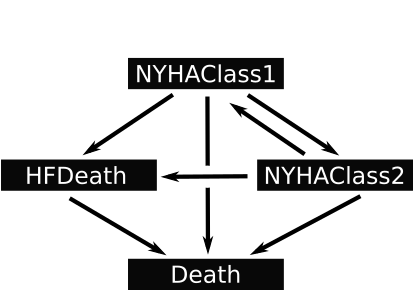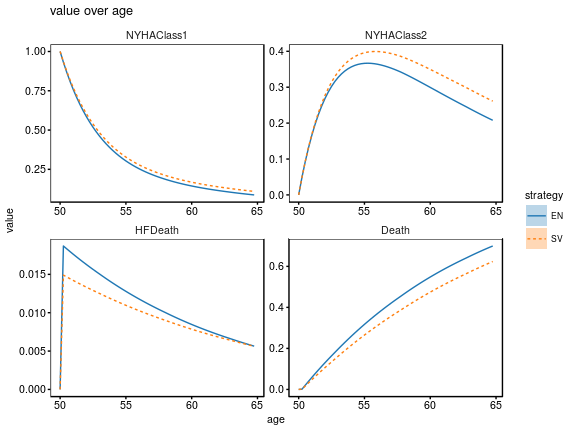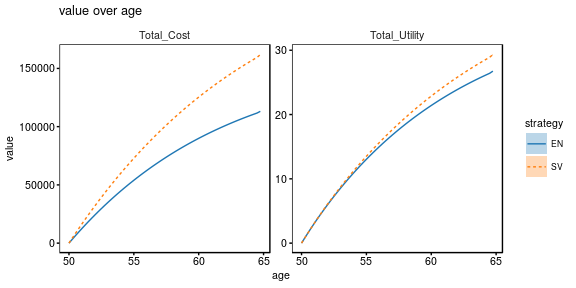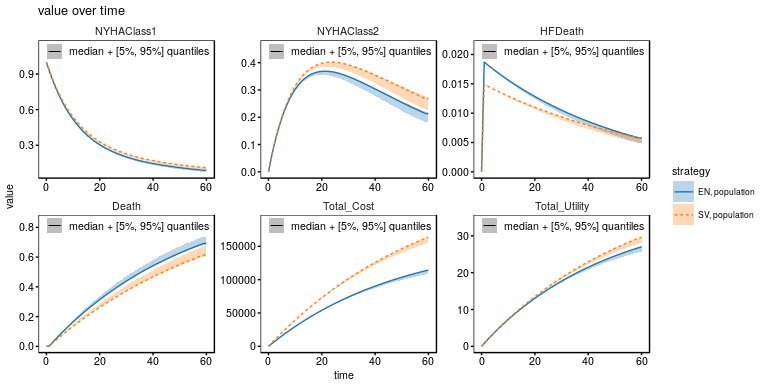# The Heart Failure example

## Background

The following model is inspired by the publication by Gaziano, Thomas A., et al. “Cost-effectiveness analysis of sacubitril/valsartan vs enalapril in patients with heart failure and reduced ejection fraction.” JAMA cardiology 1.6 (2016): 666-672.

The original Markov model with four New York Health Association (NYHA) classes has been further reduced to two classes for better suitability as illustrative example. The reduced model is visualized in the following schematics:## The mechanism of the model

The NYHA classes describe the severeness of the patient’s symptoms suffering from heart failure. Over time, symptoms can get more or less severe, indicated by the transitions between NYHAClass1 and NYHAClass2. Patients of any NHYA class can die from heart failure (HFDeath) or due to other reasons (Death). In the model, the state HFDeath turns into Death within one Markov step.

# Model set-up

For modeling with the RHEM package we need to load it with library(). The dplyr package is also useful.

library(RHEM)
library(dplyr)

In the model, the following look-up table will be evaluated:

CDC_other_cause_mortality <- data.frame(
age = seq(50, 100, by = 5),
value = c(0.0009, 0.0014, 0.0021, 0.0031, 0.0047, 0.0071, 0.0107, 0.0157, 0.0228, 0.0332, 1)
)

Note: Look-up tables will in future be part of the model definition, similar to Function()s.

## Model-building functions

A Markov model in the RHEM framework is set up step by step. Several elements like States(), Parameters(), Transition() and Value(), typically representing a Markov model, are put together by the + operator. In addition, the Markov model can and sometimes must be extended by additional Conditions(), Observations() and Events().

### States

For the example model, the states are initialized with one patient in NYHA class 1. Initially, NYHAClass2, HFDeath and Death are zero. Furthermore, conditions age (patient’s age) and year (simulation time in years) are added to the model:

markov <-
States("Model_states", NYHAClass1 = 1, NYHAClass2 = 0, HFDeath = 0, Death = 0) +
Conditions("Patient", age = 50, year = 0)

### Parameters

Parameters describe values that are constant over time. Same as for states and conditions, parameters can be attributed to different categories. In our example the parameters read:

markov <- markov +
Parameters("Transitions",
Parameters("Costs",
hosp_cost = 10698, readmission_cost = 11361) +
Parameters("Utility",
class1_util = 0.815, class2_util   = 0.72, hosp_util_dec = 0.1) +
Parameters("Enalapril", strategy = "EN",
drug_death        = 0.0187,
drug_HF_hosp_prob = 0.0344,
drug_cost_3month  = 70) +
Parameters("Sacubitril/Valsartan", strategy = "SV",
drug_death        = 0.0149,
drug_HF_hosp_prob = 0.026488,
drug_cost_3month  = 1140)

The structure of the Parameters() function is identical to States() and Conditions(). Values can either be explicitely defined as Continuous() or as raw numbers. Here, we introduce two strategies (EN and SV). The probabilities drug_death and drug_HF_hosp_prob as well as the drug costs over three months drug_cost_3month are specified per strategy. Note: The handling of strategies will in the future be simplified.

### Transitions

Transitions between Markov states are defined using the Transition() function. The name of the transition can be referred to when defining Value(). The other arguments are from (source state), to (target state) and prob (transition probability). In the example, other_cause_death is a probability that explicitely depends on age. These kinds of variables can be inline defined at the first occurrence and then be reused throughout all transitions.

markov <- markov +
Transition("v01", "NYHAClass1", "NYHAClass1",
(1 - drug_death -
(other_cause_death = Evaluate("CDC_other_cause_mortality", age))
) * 0.95) +
Transition("v02", "NYHAClass1", "NYHAClass2", (1 - drug_death - other_cause_death) * 0.05) +
Transition("v03", "NYHAClass1", "HFDeath"   ,  drug_death                                ) +
Transition("v04", "NYHAClass2", "NYHAClass1", (1 - drug_death - other_cause_death) * 0.02) +
Transition("v05", "NYHAClass2", "NYHAClass2", (1 - drug_death - other_cause_death) * 0.98) +
Transition("v06", "NYHAClass2", "HFDeath"   ,  drug_death                                ) +
Transition("v07", "NYHAClass1", "Death"     ,  other_cause_death) +
Transition("v08", "NYHAClass2", "Death"     ,  other_cause_death) +
Transition("v09", "HFDeath"   , "Death"     ,  1) +
Transition("v10", "Death"     , "Death"     ,  1)

### Values

Values, i.e. health and economic values, can be associated with Markov states or transitions. The Value() function allows to define a value by specifying an expression for each contributing state: Value("myvalue", state1 = expr1, ..., stateN = exprN). Finally, the value is computed by myvalue(t) = state1(t)*expr1 + ... + stateN(t)*exprN. In addition to states, also fluxes can be used, being referred to by the transition name. In that case, expressions are multiplied by the current flux and summed up.

markov <- markov +
Value("Utility.3months",
NYHAClass1 = class1_util - drug_HF_hosp_prob * hosp_util_dec,
NYHAClass2 = class2_util - drug_HF_hosp_prob * hosp_util_dec) +
Value("DrugCost.3months",
NYHAClass1 = drug_cost_3month,
NYHAClass2 = drug_cost_3month) +
Value("HospitalizationCost.3months",
NYHAClass2 = drug_HF_hosp_prob * hosp_cost + readmission_prob * readmission_cost)

### Markov program

In the current stage of the RHEM framework, the Markov model is automatically extended by a small “program” of events to make the Markov model executable for the DICE simulation engine. The program schedules the evaluation of Markov fluxes (evaluate_fluxes) at the next time point after start. Upon evaluation of the fluxes, the transitions are scheduled (evaluate_transitions) for the same time point and the next evaluation of fluxes is scheduled for the next time step. Auxiliary conditions like age and year should be updated when the transitions are evaluated.

We therefore add the event evaluate_transitions to the current model explicitly stating the update of age and year. When this event with an already existing name is added to a model, the event statements will be merged.

Hint: We use the evaluate_transitions event to update time-dependent conditions The same mechanism can be used to deal with time-dependent parameters which have to be introduced as conditions and need to be updated during evaluation of the transitions.

The values defined in the Value() function are the current values per time step. The Observation() function is used to accumulate these values over time, yielding the total utility and cost.

markov <- markov +
Event("evaluate_transitions",
age = . + 0.25, year = . + 0.25) +
Observation("Summary",
Cost.3months = DrugCost.3months + HospitalizationCost.3months,
Total_Utility = Accumulate(Utility.3months, time),
Total_Cost = Accumulate(Cost.3months, time))

## The Model() function

The RHEM framework provides a Model() function to set up both DICE and Markov models. The Model() function has mandatory arguments name, author, date and note to define a short name of the model, state author and date of creation and provide a detailed description of the model’s purpose. Finally, the model itself is defined within the dots argument using combining model-building functions. In this vignette, the model construction was already done before, yielding the object markov. Usually, the concatenation of conditions, parameters, events, rules and observations can be done within the dots argument.

model <- Model(
name = "Heart Failure",
author = "Daniel Kaschek",
date = "14 May 2018",
note = "A simplified Markov model of Heart Failure.",
markov
)

# Analysis set-up

Each analysis follows the same structure: Simulate(), Compare() and Plot() are concatenated by the pipe operator from the dplyr package. The purpose of the individual analysis is further specified by the arguments of the Simulate() and Compare() function.

## Comparison of states over time between the two strategies

For the first analysis, the model is simulated within the time frame 0 to 60. The comparison involves the model states, i.e. names(model$conditions$Model_states) over age instead of time. Finally, the comparison result is plotted.

model %>% Simulate(0:60) %>% Compare(names(model$conditions$Model_states), over = "age") %>% Plot()The same type of analysis is repeated for the cost and utility outcomes.

model %>% Simulate(0:60) %>% Compare(c("Total_Cost", "Total_Utility"), over = "age") %>% Plot()## Simulating a group of mixed age

The group is defined using the Sample() function. The sample name is combined with the strategies to a new strategy identifier. The dots argument of Sample() allows to define samples for each model state or condition.

simulation <-
model %>%
Simulate(0:60,
group = Sample("population",
age = rnorm(n = 10, mean = 50, sd = 10)
))

simulation %>%
Compare(c("NYHAClass1", "NYHAClass2", "HFDeath", "Death", "Total_Cost", "Total_Utility")) %>%
Plot()